# PSAT Math : How to find the solution to a binomial problem

## Example Questions

### Example Question #11 : Binomials

Multiply the binomial.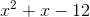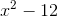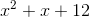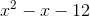By multiplying with the foil method, we multiply our first values giving, our outside values giving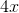. our inside values which gives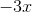, and out last values giving.